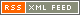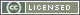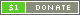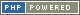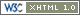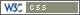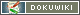# Logical Operators

Logical Operators perform logical comparisons on the terms specified, and return either `false` or the term that made the comparison true.

Symbol Type Name Description Example
High precedence (tight)
&& Infix AND True if both terms equate to `true` ` a && b `
|| Infix OR True if either term equates to `true` ` a || b `
^^ Infix XOR True if either term, but not both, equates to `true` ` a ^^ b `
! Prefix NOT True if the term is not `true` ` !a `
|?| Infix Existence True if the left term exists ` a |?| b `
Low precedence (loose)
and Infix AND True if both terms equate to `true` ` a and b `
or Infix OR True if either term equates to `true` ` a or b `
xor Infix XOR True if either term, but not both, equates to `true` ` a xor b `
not Prefix NOT True if the term is not `true` ` not a `
err Infix Existence True if the left term exists ` a err b `

Be aware that if the comparison equates to true, Boolean `true` is not returned, but the term that made the expression true. If an explicitly Boolean result is required, the Bitwise Boolean operators should be used, or else the result, or terms, should be cast to Boolean.

The logical AND, OR, and ERR Operators "short-circuit" - that is, they evaluate only as much as they need to. In the case of AND, if the first term equates to false, false will be returned without evaluating the second term. In the case of OR, if the first term is true, the first term will be returned without evaluating the second term. In the case of ERR, if the first term exists, the second term will not be evaluated.

No other operators short-circuit, so in the case of wanting an actual Boolean value to be returned by a logical comparison, bitwise Boolean comparison should not be used. Instead, the result (or the tersm) should be cast to Boolean.

Unlike most other Operators, the Logical ERR Operator - `|?|` and `ERR` - cannot be chained. So, whereas the expression `a && b && c` is valid, `a |?| b |?| c` is not.